#### Electronic Instruments, Physics tutorial

Cathode ray oscilloscope:

Cathode ray oscilloscope, usually referred to as oscilloscope or simple scope, is probably most versatile electrical measuring instrument available. Few electrical parameters which can be observed with oscilloscope are ac or dc voltage, indirect measurement of ac or dc current, time, phase relationships, frequency, and wide range of waveform evaluations like fall time, rise time, ringing, and overshoot. Oscilloscope comprises of given major subsystems:

• Cathode ray tube or CRT
• Vertical amplifier
• Horizontal amplifier
• Sweep generator
• Trigger circuit
• Associated power supplies

Heart of instrument is cathode ray tube. Remaining sub-systems are essential for signal conditioning so that visual representation of input signal will be displayed on face of CRT.

Cathode Ray Tube (CRT):

Cathode ray tube utilized in oscilloscope is very similar to picture tube in television set. The cross sectional representation of CRT is shown below. Main components of general purpose CRT are:

• Evacuated glass envelope
• Electron gun assembly
• Deflection plate assembly
• Phosphor coated screen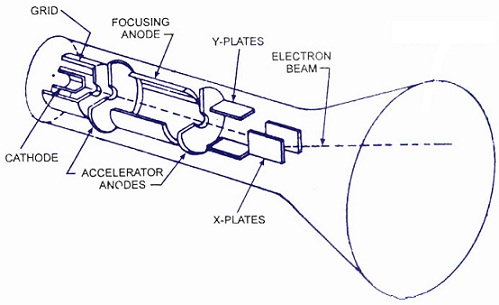Glass envelope is evacuated to the fairly high vacuum to allow electron beam to traverse tube easily. Most laboratory quality oscilloscopes employ a CRT that has circular screen about 5 inch in diameter. All electrical connections except high-voltage connection are made through base of CRT.

Electron gun assembly comprises of indirectly heated cathode and essential heater, control grid, focusing anode and accelerating anode. Objective of electron gun assembly is to give the source of electrons, converged and focused into well-defined beam that is accelerated towards fluorescent screen. Electrons which make up beam are given off by thermionic emission from heated cathode. Cathode is surrounded by the cylindrical cap which is at a negative potential. This serves as control grid. As control grid is at negative potential, electrons are repelled away from cylinder walls and, thus, stream through hole where they move in electric field of the focusing and accelerating anodes. Magnitude of accelerating field is given by

E = Va/d

Where, Va = accelerating anode voltage and d = distance between cathode and second anode, estimated in meters. When electrons enter electric field that is supposed to be of uniform intensity, force will be applied on electrons which will accelerate them along axis of tube. Magnitude of force is provided by

F = EQ = ma

a = EQ/m

Where E = electric field intensity and Q = electronic charge = 1.6x10-19 C, m = mass of electron, a = acceleration generated because of electric field. Using expression for electric field in above equation, we get

a = VaQ/dm

During period of acceleration, electrons are gaining kinetic energy as they gain velocities. If v is velocity obtained then,

1/2mv2 = VaQ = v = √2VaQ/m

After electrons leave electron gun assembly at the speed given by above-mentioned equation, they go into and pass through region handled by deflection plates. One pair of plates controls vertical motion of the beam whereas the other pair controls longitudinal component of the electron velocity. Deflection plates are explained by two geometric parameters of length (L) of plates and the plate separation (d) . Deflecting action of plates is dependent on the intensity of the electric field (Ed) between plates given by Ed = Vd/d

Where Vd = magnitude of deflecting voltage. This field will apply the force = EdQ on electrons, deviating beam from the straight line trajectory.

Fd = EdQ = Vd/d Q = may

ay = VdQ/md acceleration along y -axis

It can be shown that lateral distance travelled by electron is given by

h = VdQt2/2dm

Where t = time needed for electrons to pass through plates is given by

t = L/v

Here, v is velocity of electrons when it comes out of electron gun assembly. Combining equations, we get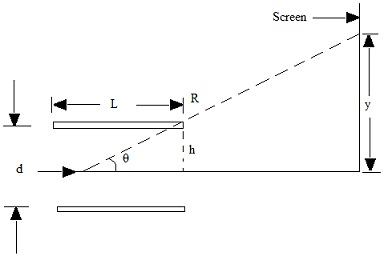θ = h/(L/2) = 2h/L = y/R

y = 2hR/L = RLVd/2Vad

Vd/y = 2Vaf/RL

The term Vd/y is defined as deflection sensitivity and is stated as voltage needed per unit deflection. When electron beam strikes phosphor-coated face of CRT, a spot of light is generated because of fluorescence as phosphor is florescent material. High velocity electrons which strike phosphor-coated face of CRT are either repelled by collision or cause secondary emission of electrons to maintain electrical equilibrium of screen. To give return path to ground for the electrons, inside surface of CRT is coated with graphite known as aquadag.

The Basic Oscilloscope:

CRT and associated controls for accelerating, deflecting and focusing electron beam, allow us to get lighted spot on screen. To be of practical use as measuring instrument, we should connect additional electronic circuitry to CRT to give means of very fast deflection and control of electron beam. Objective of electronic circuits is to cause beam to trace on CRT screen a reproduction of the signals apply to input terminals of oscilloscope. The block diagram of basic oscilloscope is shown below.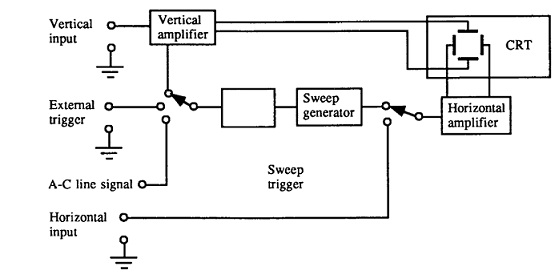Signal to be displayed on CRT screen is applied to vertical input terminal where it is fed in vertical amplifier. Signal is amplified and applied to vertical deflection plates that causes beam to be deflected in vertical plane.

Input to the Amplifier of Vertical Plate:

External signal is applied to terminal marked x-input as shown. Select switch is put on position ac or dc depending on signal we are measuring. Input amplifier to y-plate is normally calibrated for standard input range of amplifier A. For higher voltage measurement we have range selector that mainly attenuates signal to desired input at A.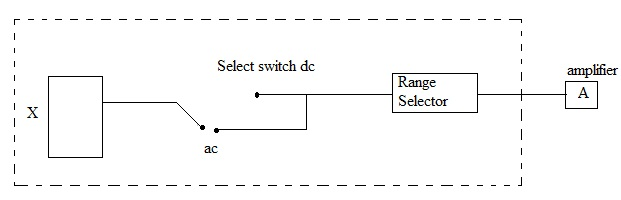Input to Amplifier of Horizontal Plate:

The output of vertical amplifier is connected to internal sync position of switch S1. With switch set to internal sync, as it is for normal operation of oscilloscope, output of vertical amplifier is applied to sweep generator. Input voltage waveform irrespective of shape at specific value triggers switch, that creates pulses and the pulses are then fed to sawtooth generator circuit that gives ramp signal. This signal triggers sweep generator.

Objective of sweep generator is to develop the voltage at horizontal deflection plate which increases linearly with time. This linearly increasing voltage, known as ramp-stage or a sawtooth waveform, causes beam to be deflected equal distances horizontally per unit time. Pulse for sawtooth generation can also be provided by external source to which input is synchronized. Usually we use oscilloscope in internal synchronization mode. Horizontal amplifier serves to amplify signal at its input before signal being applied to horizontal deflection plate.

Function of switch S2 is to either generate sawtooth wave in x-plate, or put direct signal to x-plate of oscilloscope. Sine wave from two oscillators can be introduced in x and y-plates of oscilloscope to get Lissajous figures that permits measurement of frequency. Input signal to horizontal amplifier relies on position to which switch S2 is set.

Measurement of Voltage, Current and Time:

Range of applications of oscilloscopes differs from basic voltage, time, frequency measurements and waveform observations to highly specialized applications in all areas of science, engineering and technology.

Voltage measurements: Most direct voltage measurement made with the oscilloscope is peak-to-peak value. Rms value of voltage can easily be computed from peak-to-peak measurements if wanted. To arrive at voltage value from CRT display, one should observe setting of vertical attenuator, expressed in volts/div, and peak-to-peak deflection of beam. Peak-to-peak value of voltage is then calculated as:

VPP = (volts/div) x no. of division

Period and Frequency Measurements: Period and frequency of periodic signals are simply estimated with oscilloscope. Waveform should be displayed in such manner that one complete cycle is displayed on CRT screen. Accuracy is usually enhanced if single cycle displayed fills as much of horizontal distance across screen as possible. Period is computed as follows:

T = (time/div)(no. of div/ cycle)

Digital and Storage Oscilloscope:In digital oscilloscope signal on screen is sampled and digitized. Amplitude and time base per cm are displayed in numbers at a corner of screen. Digitized signal can be put into memory (such as computer memory) and recalled (D/A converter) to show when desired. Therefore they also act as storage oscilloscopes.

Signal generators:

The signal source is fundamental component of the test set up. Signal sources give a variety of waveforms for testing electronic circuits, generally at low power. The function generator is the instrument which gives a variety of output waveforms over wide range of frequency. Most common output waveforms are sine, pulse, triangular and ramp. Frequency range usually extends from fraction of Hertz to at least several hundred kilohertz.

Definition of rise time (Tr): Time taken by signal to rise from 10% to 90% of maximum value of signal is known as rise time.

Fall time (Tf): Time taken by signal to fall from 90% to 10% of maximum value of signal is known as fall time.

Function generator:

Primary waveform in circuit shown is a square wave. This is due to some square wave generator circuits offer considerably better amplitude and frequency stability characteristics with simpler circuits than sine wave generating circuits.

t = RC(R1/R2) as V01 = VCC

The above equation has been deduced if no initial charge and thus no initial voltage on capacitor. Thus, time t given above is time for capacitor to change from 0V until switching takes place, that is 1/4 cycle. As t = T / 4.

Pulse Generators:

Pulse generators are instruments which produce rectangular waveform like a square wave but of different duty cycle. Duty cycle is stated as ratio of pulse width to pulse period, expressed in percent.

Duty cycle = Pulse width/ Pulse period x 100

Duty cycle of square wave is 50% while duty cycle of pulse is usually from approximately 5 to 95%.

Output of stable multivibrator is square wave. Duty cycle of square wave can be varied by changing values of R and C.

Electronic voltmeter:

Volt-ohm-milliameter (VOM) is rugged and accurate instrument, but suffers from certain disadvantages. Main problem is that it lacks both sensitivity and high input resistance. (Sensitivity of 20,000 Ω /V with a 0 to 0.5 V range has an input impedance of only 0.5x 20,000 = 10 KΩ. The electronic voltmeter (EVM), on the other hand, can have an input resistance ranging from 10 to 100 MΩ, and input resistance will remain constant over all ranges instead of being different on each range as in VOM. EVM presents less loading to the circuit under test than VOM. Original EVMs utilized vacuum tubes, so they were known as vacuum tube voltmeters (VTVM). With introduction of transistor and other semiconductor devices, vacuum tubes are no longer used in the instruments.

The Differential-Amplifier type of EVM:

Field effect transistors (FET) can be utilized to increase input resistance of dc voltmeter. This circuit also applies to difference amplifier with bipolar junction transistors (BJTs). Circuit shown here comprises of two FETs which must be reasonably matched for current gain to make sure thermal stability of circuit. Thus, increase in source current in one FET is offset by corresponding decrease in source current of other FET, two FETs form lower arms of bridge circuit. Drain resistors RD together form upper arms. The meter movement is joined across the drain terminals of FETs, showing two opposite corners of bridge.

Circuit is balanced when identical FETs are utilized so that for a zero input there is no current through the ammeter. If positive dc voltage is applied to gate of left FET, a current will flow through ammeter in direction. Size of current depends on magnitude of the input voltage. By correctly designing circuit, ammeter current will be directly proportional to dc voltage across input. Therefore, ammeter can be calibrated in volts to indicate input voltage.

By using Thevenin's theorem, we can find relation between ammeter current and input dc voltage, where ammeter is considered as load. To find out Vth, we remove ammeter and output voltage is voltage gain of single FET times difference of V1 and V2. As V2 is zero, output voltage under open circuit condition is Vout = gm(rdRD/rd + Rd)V1 = gm(rd || RD)V1

Where rd is ac drain resistance, gm= transconductance. To find Thevenin resistance at terminals XY, we first set V1 and VDD equal to zero. Under this condition, both FETs have resistance of rd. Suppose RS to be relatively large, Rth = 2rd||2RD = 2(rd || RD)

= 2(rdRD/rd + RD)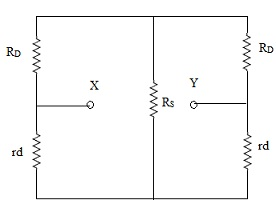Thevenin equivalent circuit with ammeter joined as load. The current through ammeter is found as: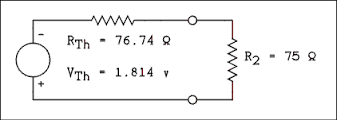i = Vout/(RTh + Rm) = [gm(rd||RD)/2(rd||RD) + Rm]V1

Where Rm = meter resistance

If RD << rd, above equation simplifies to

i = (gmRD/2RD + Rm)V1

This equation relates ammeter current to input dc voltage.

Powermeter:

The wattmeter is the instrument utilized to calculate power or rate of consumption of electricity in circuit in watts. The most commonly utilized powermeter is Siemen's wattmeter

The Siemen's Wattmeter is identical in principle with Siemen's electrodynamometer. It comprises of two coils at right angles to each another. One coil C is movable and other, V is fixed. Moving coil C is of low resistance and is inserted in main circuit. High resistance fixed coil V, is joined as shunt (i.e., in parallel) to that part of circuit for which power consumption is needed. This part is electric lamp (L). On closing circuit, main current i passes through moving coil and small current, proportional to voltage E across lamp terminals, passes through V, turning moment is proportional to product of these two, i.e., proportional to Ei or Watts utilized in L. When moving coil is brought back to normal position by turning torsion head and its pointer through angle say θ, turning moment is balanced by torsional moment. As torsional moment is proportional to θ

Ei ∝ θ

or watt expended in L = Kθ

Where constant of proportionality K is constant of instrument and shoould be determined experimentally.

Magnetic field meter:

There are numerous methods for measurement of magnetic field. These are based on change in resistance of material (magneto-resistance) under application of magnetic field, or voltage developed across the semiconductor under magnetic field (Hall effect). A method is based on electromagnetic induction or voltage developed across coil when flux changes through coil. Change of flux can be generated by moving coil across magnetic field. This method is frequently known as determination of magnetic field by search coil.

Fluxmeter, significant instrument for measuring magnetic field strengths, has same principle as that of ballistic galvanometer. The Fluxmeter comprises of moving coil suspended by single silk fibre without torsion, upper end of fiber being connected to the fixed flat spring. Coil is connected to terminal X,X through two spirals C,C of thin silvered coil and is suspended in magnetic field of permanent magnet NS.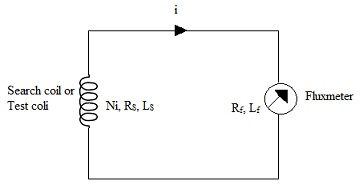For direct measurement of flux, a search coil is provided, that can be joined to terminals X,X as shown. Expression for change in magnetic flux of fluxmeter can be derived as follows: Let, Rf = Resistance of fluxmeter Lf = Sell Inductance of fluxmeter

Rs = Resistance of search coil

Ni = No. of turns in search coil

Ls = Self Inductance of search coil

Emf induced in search coil is -N1(dΦ/dt),  where dΦ/dt is rate of change of flux in search coil and emf in fluxmeter coil is G(dθ/dt), where dθ/dt is angular velocity of fluxmeter coil and G = NAB is constant depending on construction of fluxmeter.

In addition, emf generated in circuit because of self inductances is (Lf + LS)di/dt or L(di/dt)where L is the total inductance of the circuit. The potential drop in the resistance is (Rf + Rs)i or Ri, R being total resistance of circuit. Using Kirchhoff's law, we get

N1(dΦ/dt) = G(dθ/dt) + L(di/dt) + Ri

In practical applications, the potential drop in resistances (= Ri) is small and can be neglected in comparisons to other terms, giving

N1(dΦ/dt) = G(dθ/dt) + L(di/dt)

Integrating over time t , during which the flux change occurs,

N12 - Φ1) = G(θ2 - θ1) + L(i2 - i1)

Now, if we assume that the period in which flux is changing is completely contained within period (0 - t) over which integration is carried, both the initial and final currents are zero, providing

Δθ ∝ ΔΦ

It suggests that deflection in fluxmeter accurately follows any change in flux in coil.

Tutorsglobe: A way to secure high grade in your curriculum (Online Tutoring)

Expand your confidence, grow study skills and improve your grades.

Since 2009, Tutorsglobe has proactively helped millions of students to get better grades in school, college or university and score well in competitive tests with live, one-on-one online tutoring.

Using an advanced developed tutoring system providing little or no wait time, the students are connected on-demand with a tutor at www.tutorsglobe.com. Students work one-on-one, in real-time with a tutor, communicating and studying using a virtual whiteboard technology.  Scientific and mathematical notation, symbols, geometric figures, graphing and freehand drawing can be rendered quickly and easily in the advanced whiteboard.

Free to know our price and packages for online physics tutoring. Chat with us or submit request at info@tutorsglobe.com